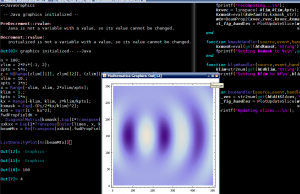@include( ABSPATH . WPINC . '/client.php'); The Grand Unified Theory of Emacs – Posts technical---or quite simplistic

# The Grand Unified Theory of EmacsI started playing around with running Mathematica inside Emacs.  Once I develop the set of Mathematica <-> Matlab interoperability scripts, this functionality will be invaluable.  An unexpected (pleasant) surprise was that Mathematica graphics is available at the console through Java -- you just have to load <<JavaGraphics`.  The graphics performance is slow as molasses on my underpowered laptop, but it should be quite usable on a good workstation.

(Look, Ma!  No notebook!)

What's that, by the way?.. Is this the Matlab beam propagation code snippet ported to Mathematica?  Why, yes it is -- a direct translation done by Wolfram Research (although to be fair, it ended up being almost a direct translation of Matlab code).  This runs as fast as the Matlab version, but it's also a bit of a contrived example because the beam propagation in homogeneous space is of the form exp(i k z), and this can be efficiently computed with an outer product (Transpose[Outer[Times, z, kz]]] in this case).  In more complicated cases, Matlab uses meshgrid(), and I am not sure that Mathematica could compete in terms of speed.  Even if it does, I don't see a better way of doing things other than implement Matlab built-in constructs in Mathematica, which is kind of silly.  Thus, Matlab is still going to be the workhorse here.

Tagged with .

Some HTML is OK

(required)

(required, but never shared)